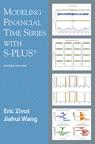# Modeling Financial Time Series with S-PLUS®, Second Edition

Home
News
Contents
Scripts
Errata
Additions
S+FinMetrics
Order# Contents

The field of financial econometrics has exploded over the last decade. This book represents an integration of theory, methods, and examples using the S-PLUS statistical modeling language and the S+FinMetrics™ module to facilitate the practice of financial econometrics. This is the first book to show the power of S-PLUS for the analysis of time series data. It is written for researchers and practitioners in the finance industry, academic researchers in economics and finance, and advanced MBA and graduate students in economics and finance. Readers are assumed to have a basic knowledge of S-PLUS and a solid grounding in basic statistics and time series concepts.

## Overview of the Book

The chapters in the book cover univariate and multivariate models for analyzing financial time series using S-PLUS and the functions in S+FinMetrics™. Chapter one gives a general overview of the use of S-PLUS 6 and highlights certain aspects of the language for statistical modeling. Chapter two introduces the new time series objects in S-PLUS 6 and illustrates the specification, manipulation and visualization of these objects. Chapter three surveys time series concepts used throughout the book. Chapters four through eight cover a variety of topics in the modeling of univariate financial time series, including testing for unit roots, extreme value theory, time series regression models, GARCH models of volatility, and long memory models. Chapter nine introduces rolling analyses of time series models and covers related topics such as technical analysis of financial time series and moving average methods for high frequency data. Chapters ten through fifteen cover models for the analysis of multivariate financial time series. Topics include systems of regression equations, classical and Bayesian vector autoregressive models, cointegration, factor models, multivariate GARCH models, and state space models. Chapter 16 covers aspects of modeling time series arising from fixed income financial assets. Chapter 17, written by Victor Yohai and Jiahui Wang, describes robust REGARIMA models that allow for structural change. Chapters 18 through 23 are new to the Second Edition of the book. These new chapters cover nonlinear regime-switching models, copulas, continuous-time models, the generalized method of moments, semi-nonparametric conditional density models, and the efficient method of moments.

## Table of Contents

Abbreviated Table of Contents

S and S-PLUS * Time Series Specification, Manipulation and Visualization in S-PLUS * Time Series Concepts * Unit Root Tests * Modeling Extreme Values * Time Series Regression Modeling * Univariate GARCH Models * Long Memory Time Series Modeling * Rolling Analysis of Time Series * Systems of Regression Equations * Vector Autoregressive Models for Multivariate Time Series * Cointegration * Multivariate GARCH Modeling * State Space Models * Factor Models for Asset Returns * Term Structure of Interest Rates * Robust Change Detection * Nonlinear Models * Copulas * Continuous Time Financial Models * Generalized Method of Moments * Seminonparametric Conditional Density Models * Efficient Method of Moments * Index

Full Table of Contents

Last updated: February 13, 2006